# 16 VECTOR CALCULUS VECTOR CALCULUS 16 9 The

• Slides: 52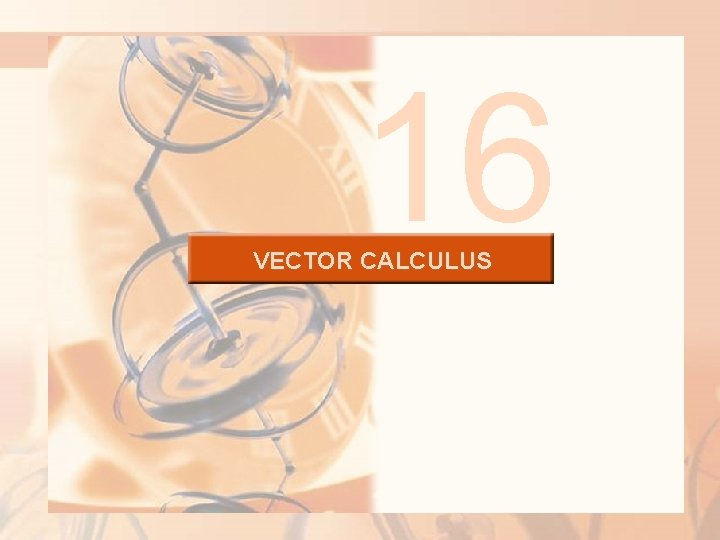16 VECTOR CALCULUS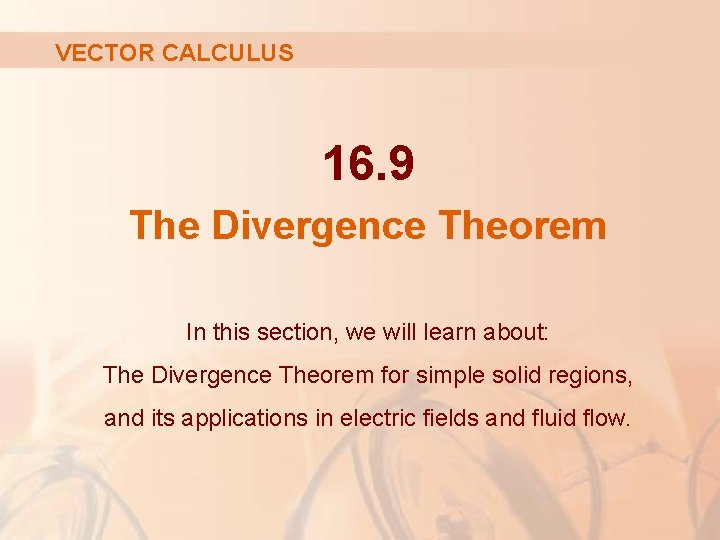VECTOR CALCULUS 16. 9 The Divergence Theorem In this section, we will learn about: The Divergence Theorem for simple solid regions, and its applications in electric fields and fluid flow.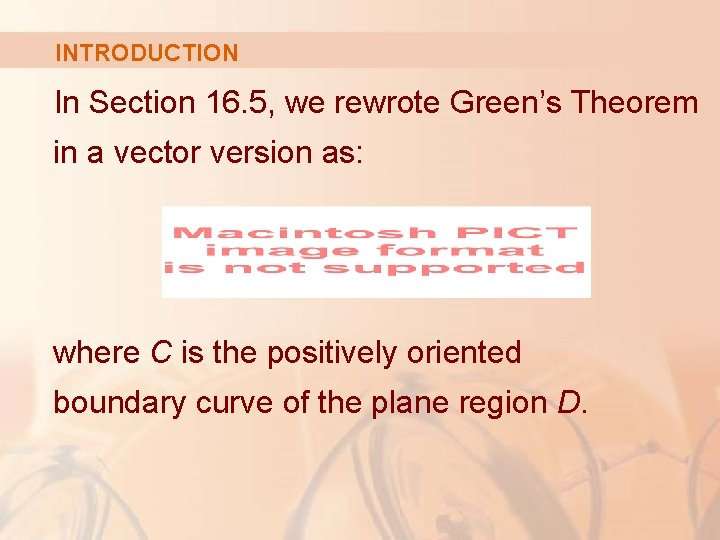INTRODUCTION In Section 16. 5, we rewrote Green’s Theorem in a vector version as: where C is the positively oriented boundary curve of the plane region D.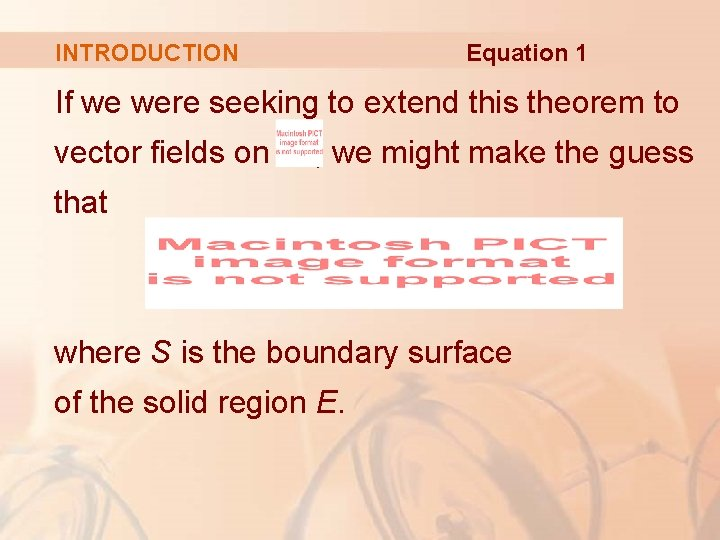Equation 1 INTRODUCTION If we were seeking to extend this theorem to vector fields on , we might make the guess that where S is the boundary surface of the solid region E.DIVERGENCE THEOREM It turns out that Equation 1 is true, under appropriate hypotheses, and is called the Divergence Theorem.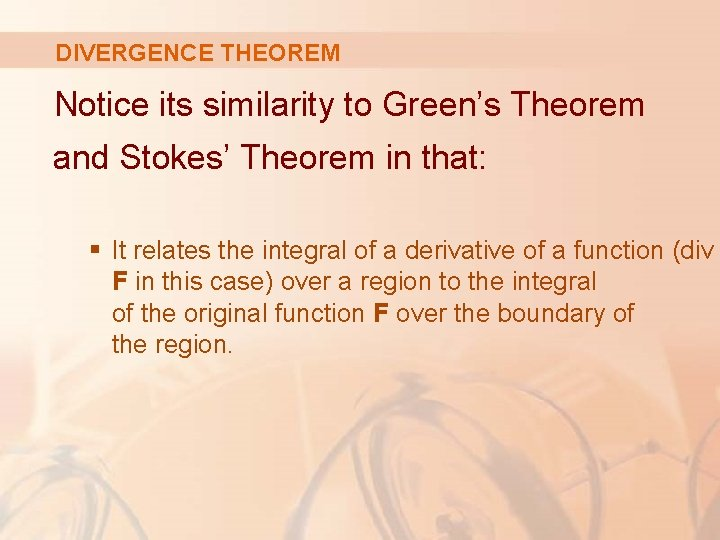DIVERGENCE THEOREM Notice its similarity to Green’s Theorem and Stokes’ Theorem in that: § It relates the integral of a derivative of a function (div F in this case) over a region to the integral of the original function F over the boundary of the region.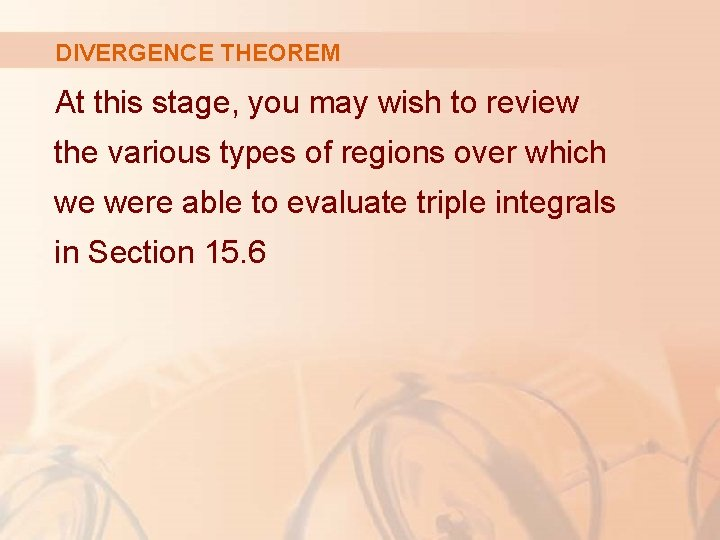DIVERGENCE THEOREM At this stage, you may wish to review the various types of regions over which we were able to evaluate triple integrals in Section 15. 6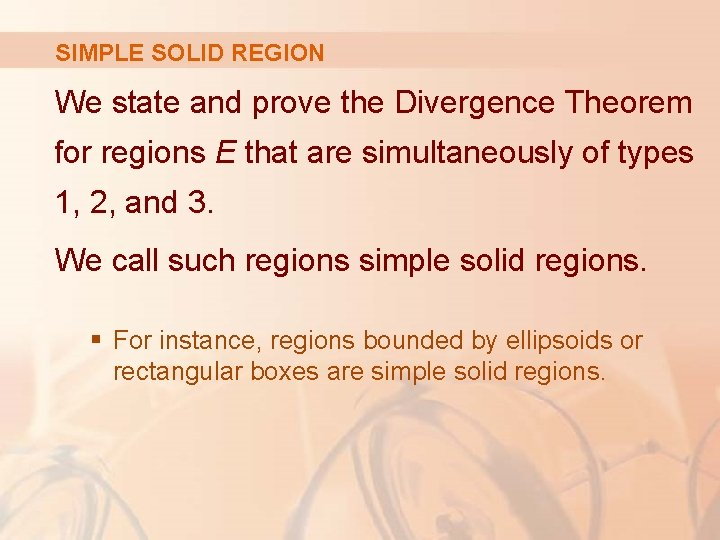SIMPLE SOLID REGION We state and prove the Divergence Theorem for regions E that are simultaneously of types 1, 2, and 3. We call such regions simple solid regions. § For instance, regions bounded by ellipsoids or rectangular boxes are simple solid regions.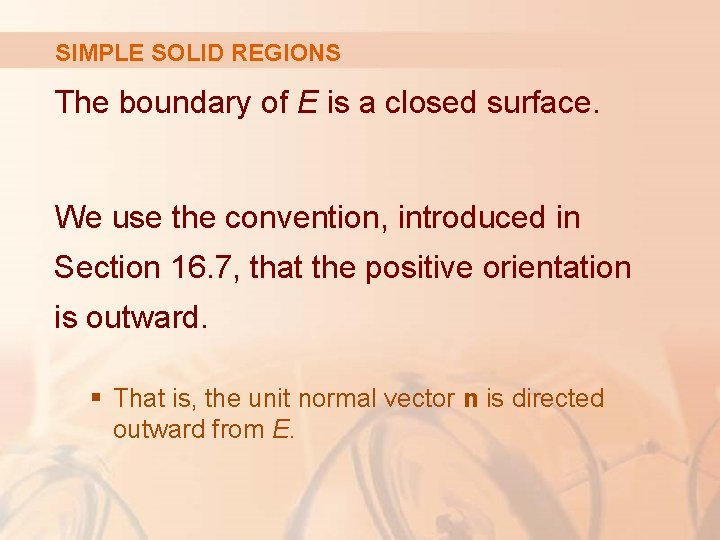SIMPLE SOLID REGIONS The boundary of E is a closed surface. We use the convention, introduced in Section 16. 7, that the positive orientation is outward. § That is, the unit normal vector n is directed outward from E.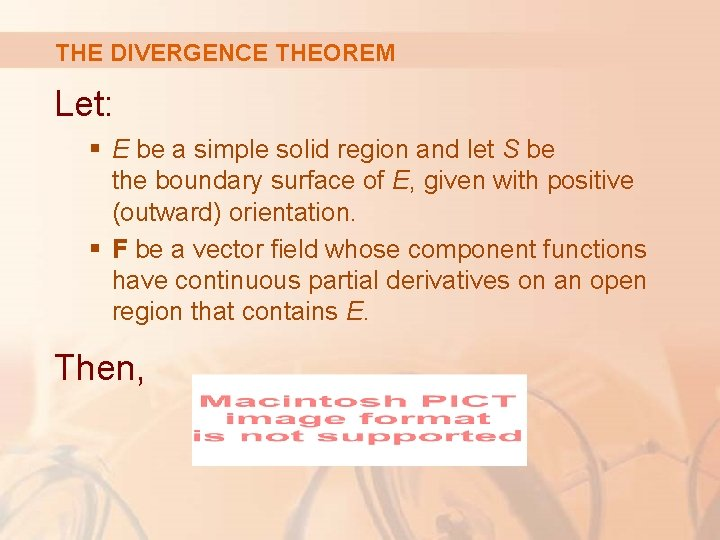THE DIVERGENCE THEOREM Let: § E be a simple solid region and let S be the boundary surface of E, given with positive (outward) orientation. § F be a vector field whose component functions have continuous partial derivatives on an open region that contains E. Then,THE DIVERGENCE THEOREM Thus, the Divergence Theorem states that: § Under the given conditions, the flux of F across the boundary surface of E is equal to the triple integral of the divergence of F over E.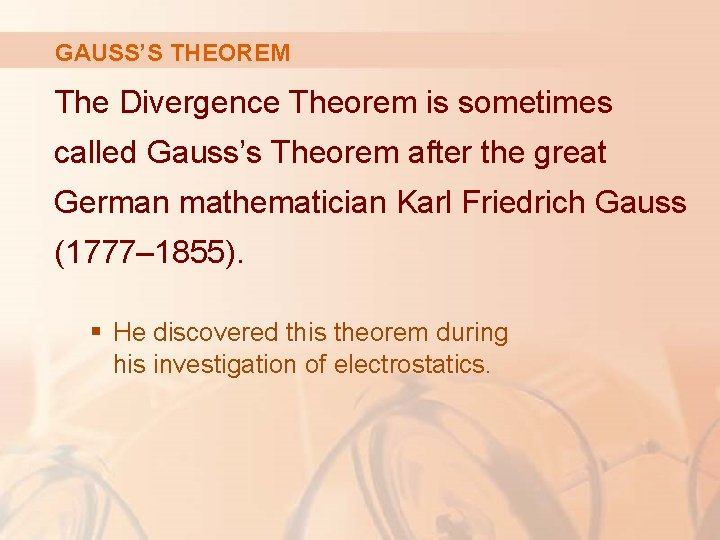GAUSS’S THEOREM The Divergence Theorem is sometimes called Gauss’s Theorem after the great German mathematician Karl Friedrich Gauss (1777– 1855). § He discovered this theorem during his investigation of electrostatics.OSTROGRADSKY’S THEOREM In Eastern Europe, it is known as Ostrogradsky’s Theorem after the Russian mathematician Mikhail Ostrogradsky (1801– 1862). § He published this result in 1826.THE DIVERGENCE THEOREM Let F = P i + Q j + R k § Then, § Hence, Proof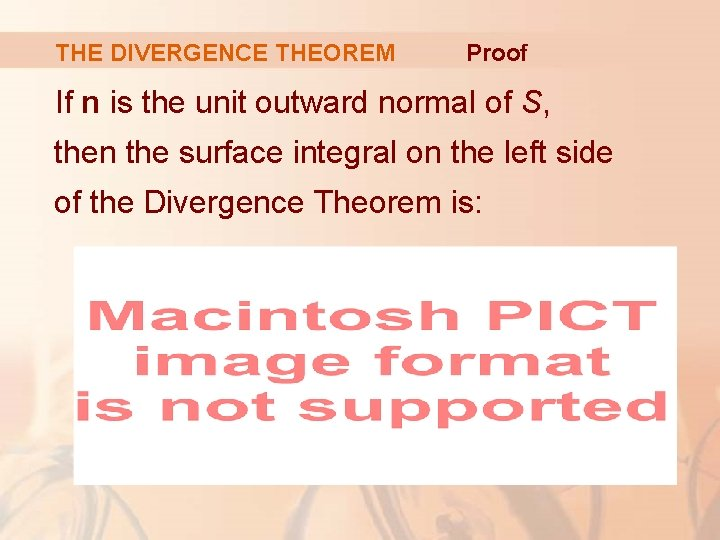THE DIVERGENCE THEOREM Proof If n is the unit outward normal of S, then the surface integral on the left side of the Divergence Theorem is: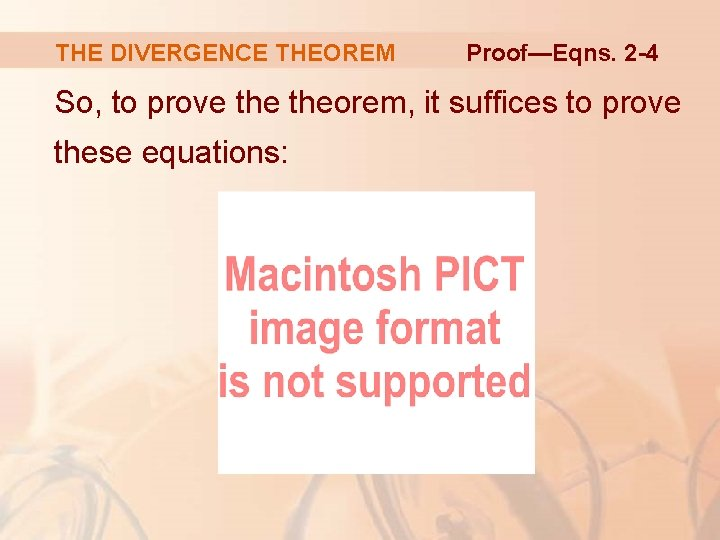THE DIVERGENCE THEOREM Proof—Eqns. 2 -4 So, to prove theorem, it suffices to prove these equations: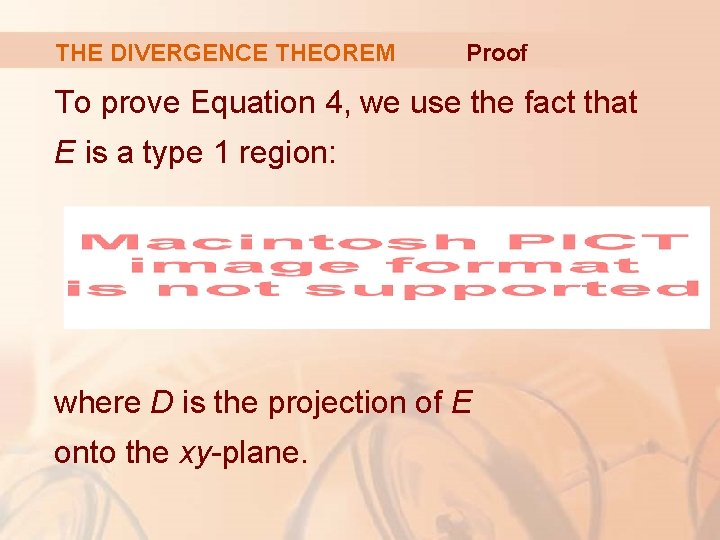THE DIVERGENCE THEOREM Proof To prove Equation 4, we use the fact that E is a type 1 region: where D is the projection of E onto the xy-plane.THE DIVERGENCE THEOREM Proof By Equation 6 in Section 15. 6, we have:THE DIVERGENCE THEOREM Proof—Equation 5 Thus, by the Fundamental Theorem of Calculus,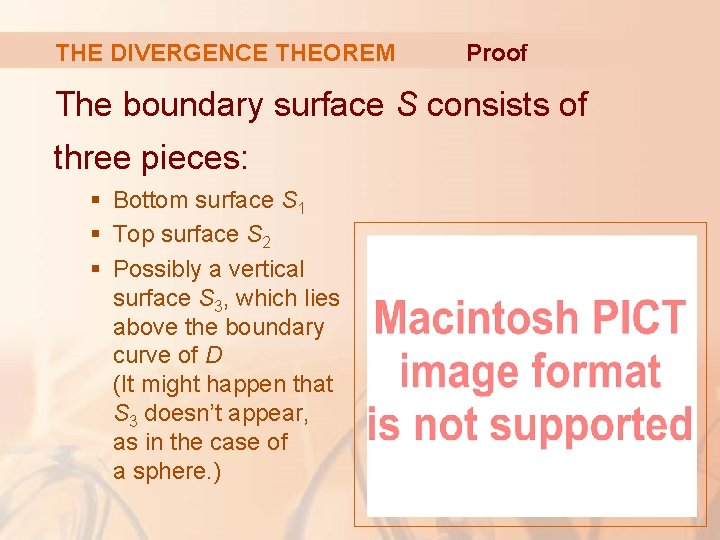THE DIVERGENCE THEOREM Proof The boundary surface S consists of three pieces: § Bottom surface S 1 § Top surface S 2 § Possibly a vertical surface S 3, which lies above the boundary curve of D (It might happen that S 3 doesn’t appear, as in the case of a sphere. )THE DIVERGENCE THEOREM Proof Notice that, on S 3, we have k ∙ n = 0, because k is vertical and n is horizontal. § Thus,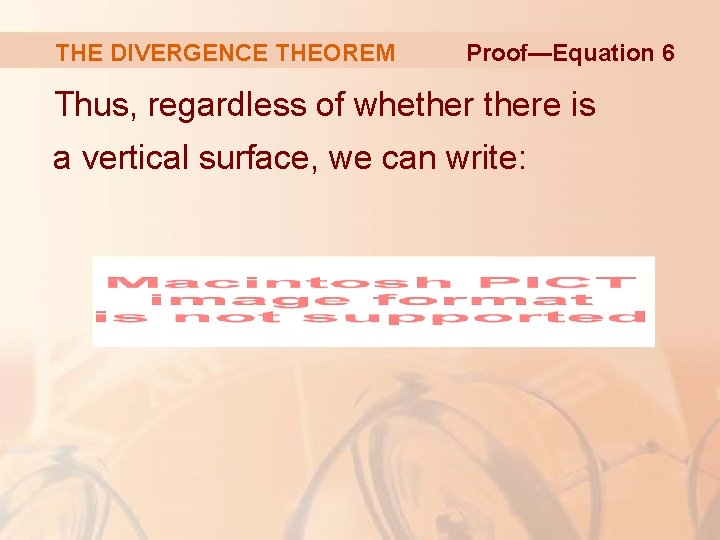THE DIVERGENCE THEOREM Proof—Equation 6 Thus, regardless of whethere is a vertical surface, we can write: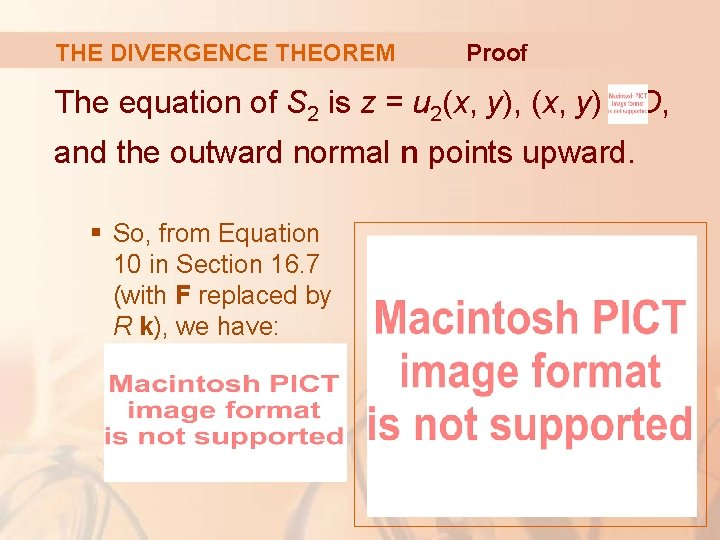THE DIVERGENCE THEOREM Proof The equation of S 2 is z = u 2(x, y), (x, y) and the outward normal n points upward. § So, from Equation 10 in Section 16. 7 (with F replaced by R k), we have: D,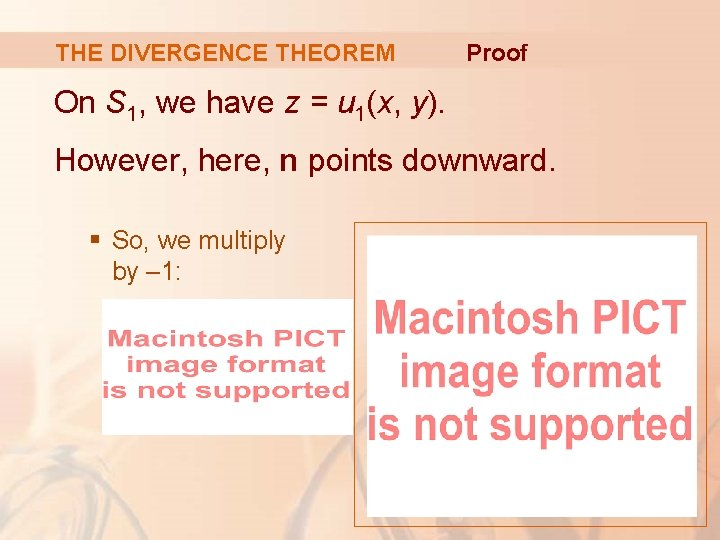THE DIVERGENCE THEOREM Proof On S 1, we have z = u 1(x, y). However, here, n points downward. § So, we multiply by – 1: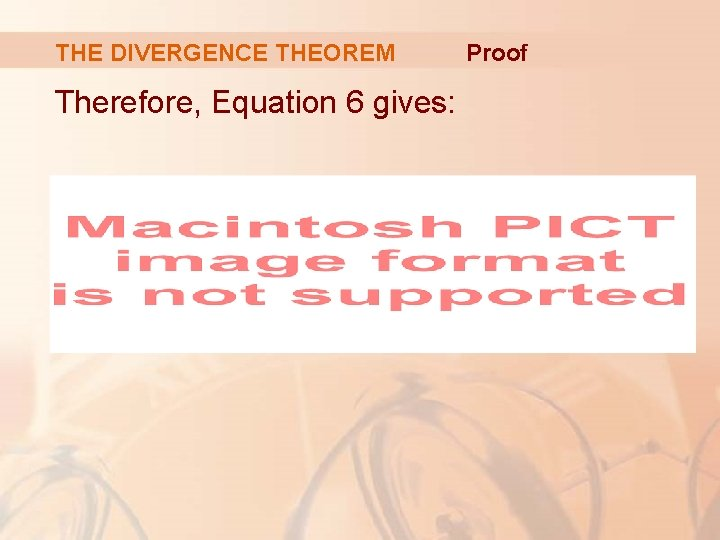THE DIVERGENCE THEOREM Therefore, Equation 6 gives: Proof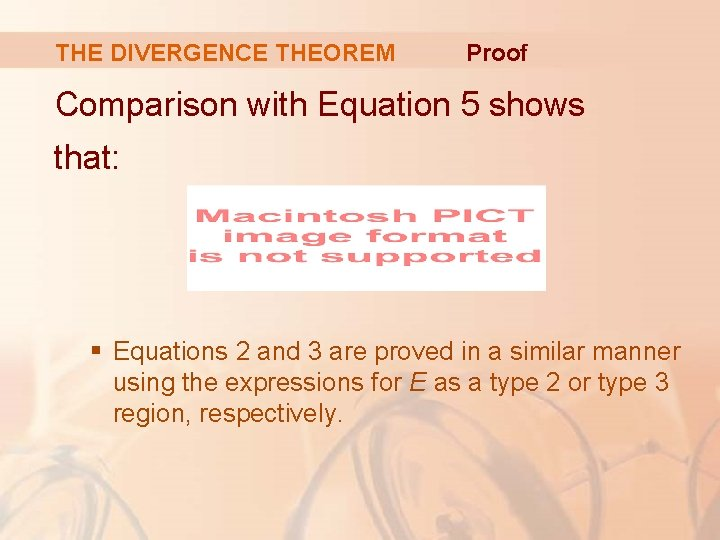THE DIVERGENCE THEOREM Proof Comparison with Equation 5 shows that: § Equations 2 and 3 are proved in a similar manner using the expressions for E as a type 2 or type 3 region, respectively.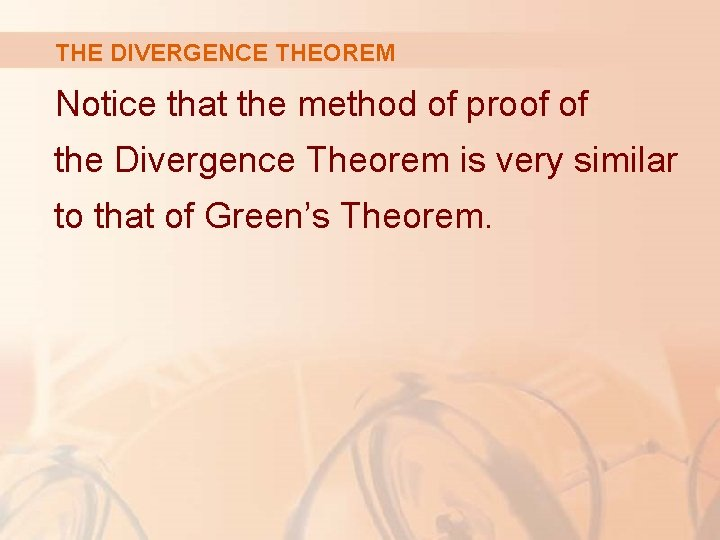THE DIVERGENCE THEOREM Notice that the method of proof of the Divergence Theorem is very similar to that of Green’s Theorem.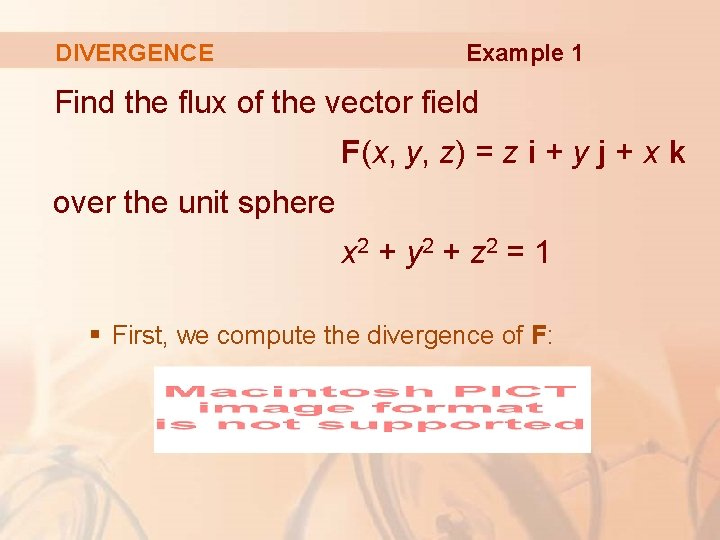DIVERGENCE Example 1 Find the flux of the vector field F(x, y, z) = z i + y j + x k over the unit sphere x 2 + y 2 + z 2 = 1 § First, we compute the divergence of F:DIVERGENCE Example 1 The unit sphere S is the boundary of the unit ball B given by: x 2 + y 2 + z 2 ≤ 1 § So, the Divergence Theorem gives the flux as:Example 2 DIVERGENCE Evaluate where: 2 § F(x, y, z) = xy i + (y 2 + exz ) j + sin(xy) k § S is the surface of the region E bounded by the parabolic cylinder z = 1 – x 2 and the planes z = 0, y + z = 2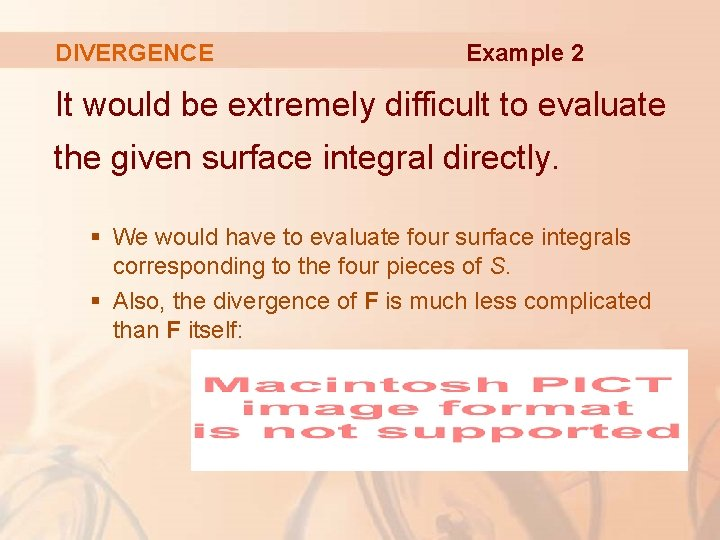DIVERGENCE Example 2 It would be extremely difficult to evaluate the given surface integral directly. § We would have to evaluate four surface integrals corresponding to the four pieces of S. § Also, the divergence of F is much less complicated than F itself: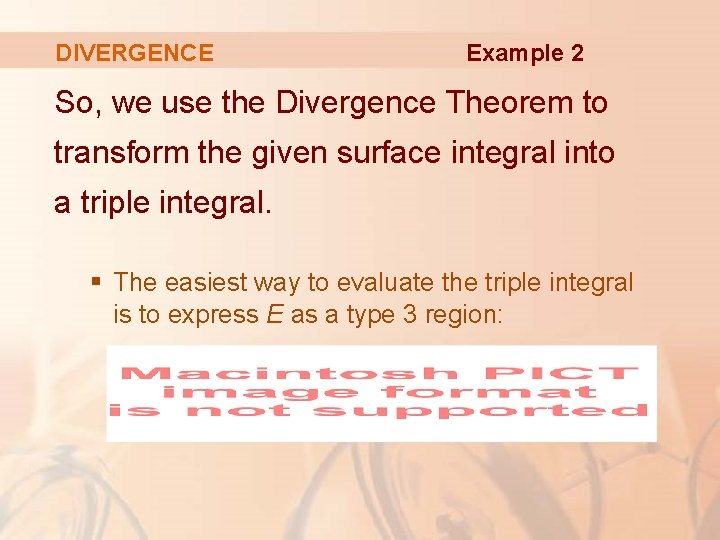DIVERGENCE Example 2 So, we use the Divergence Theorem to transform the given surface integral into a triple integral. § The easiest way to evaluate the triple integral is to express E as a type 3 region:DIVERGENCE Then, we have: Example 2DIVERGENCE Example 2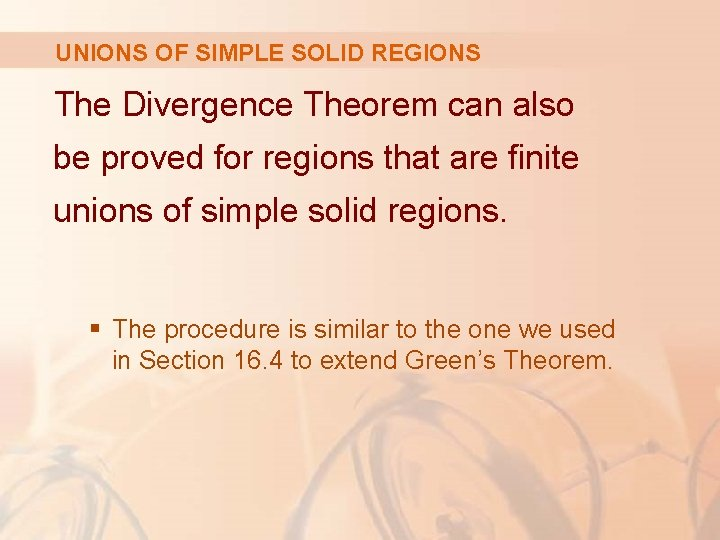UNIONS OF SIMPLE SOLID REGIONS The Divergence Theorem can also be proved for regions that are finite unions of simple solid regions. § The procedure is similar to the one we used in Section 16. 4 to extend Green’s Theorem.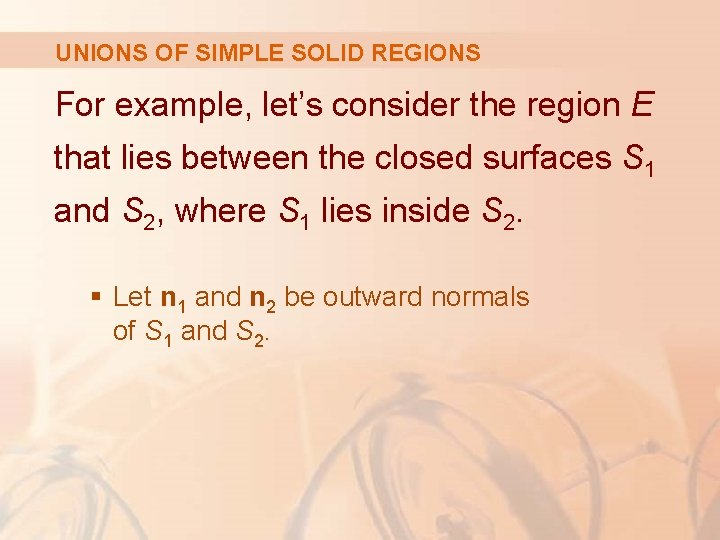UNIONS OF SIMPLE SOLID REGIONS For example, let’s consider the region E that lies between the closed surfaces S 1 and S 2, where S 1 lies inside S 2. § Let n 1 and n 2 be outward normals of S 1 and S 2.UNIONS OF SIMPLE SOLID REGIONS Then, the boundary surface of E is: S = S 1 Its normal n is given by: n = –n 1 on S 1 n = n 2 on S 2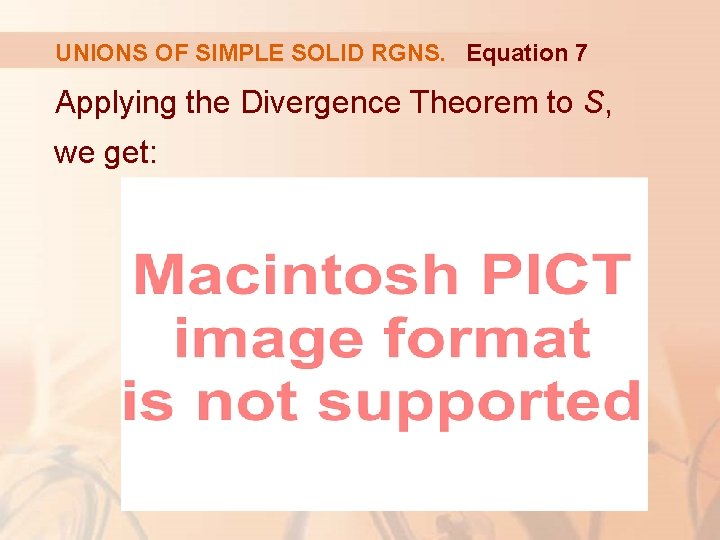UNIONS OF SIMPLE SOLID RGNS. Equation 7 Applying the Divergence Theorem to S, we get:APPLICATIONS—ELECTRIC FIELD Let’s apply this to the electric field (Example 5 in Section 16. 1): where S 1 is a small sphere with radius a and center the origin.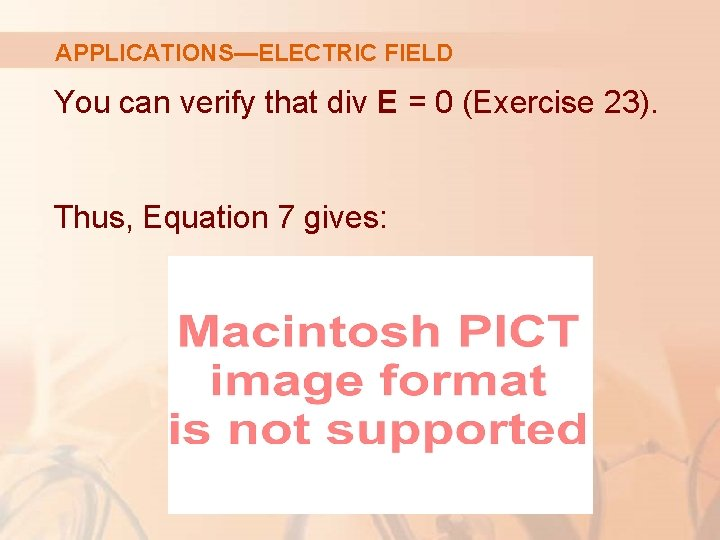APPLICATIONS—ELECTRIC FIELD You can verify that div E = 0 (Exercise 23). Thus, Equation 7 gives:APPLICATIONS—ELECTRIC FIELD The point of this calculation is that we can compute the surface integral over S 1 because S 1 is a sphere.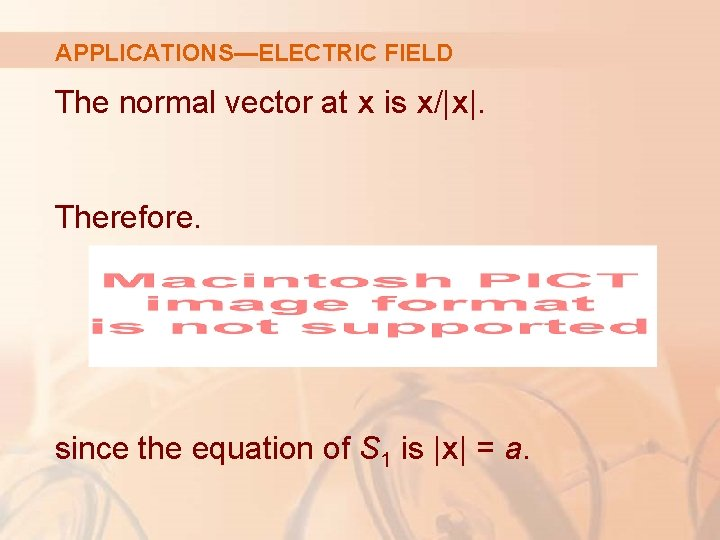APPLICATIONS—ELECTRIC FIELD The normal vector at x is x/|x|. Therefore. since the equation of S 1 is |x| = a.APPLICATIONS—ELECTRIC FIELD Thus, we have: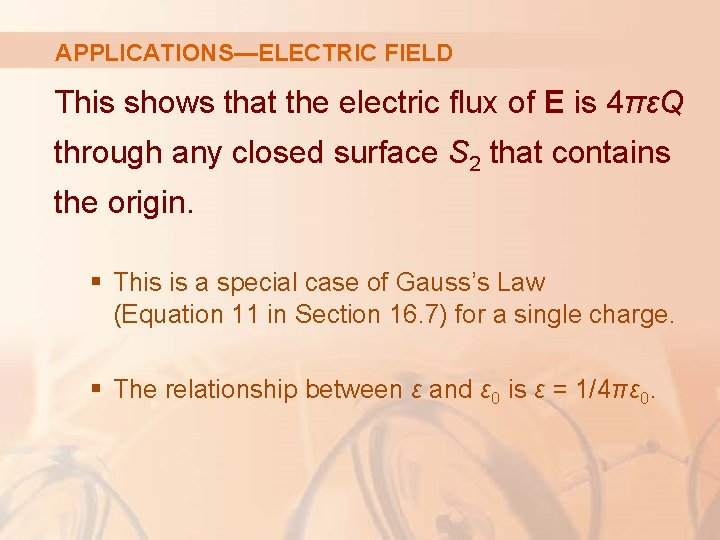APPLICATIONS—ELECTRIC FIELD This shows that the electric flux of E is 4πεQ through any closed surface S 2 that contains the origin. § This is a special case of Gauss’s Law (Equation 11 in Section 16. 7) for a single charge. § The relationship between ε and ε 0 is ε = 1/4πε 0.APPLICATIONS—FLUID FLOW Another application of the Divergence Theorem occurs in fluid flow. § Let v(x, y, z) be the velocity field of a fluid with constant density ρ. § Then, F = ρv is the rate of flow per unit area.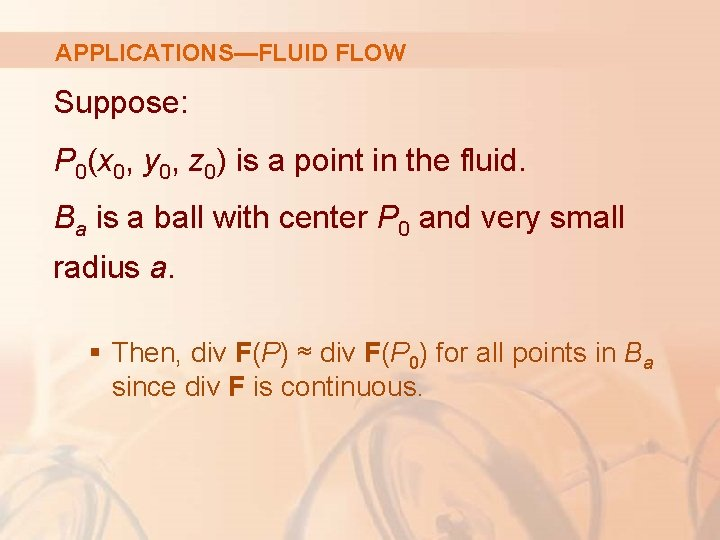APPLICATIONS—FLUID FLOW Suppose: P 0(x 0, y 0, z 0) is a point in the fluid. Ba is a ball with center P 0 and very small radius a. § Then, div F(P) ≈ div F(P 0) for all points in Ba since div F is continuous.APPLICATIONS—FLUID FLOW We approximate the flux over the boundary sphere Sa as follows: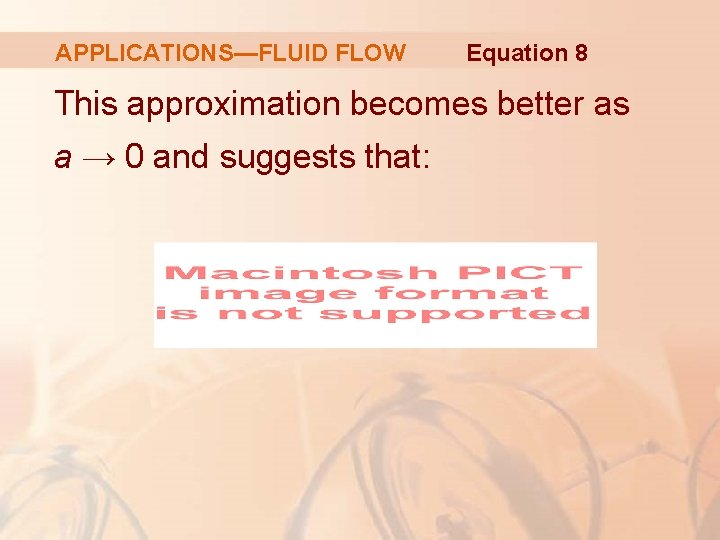APPLICATIONS—FLUID FLOW Equation 8 This approximation becomes better as a → 0 and suggests that: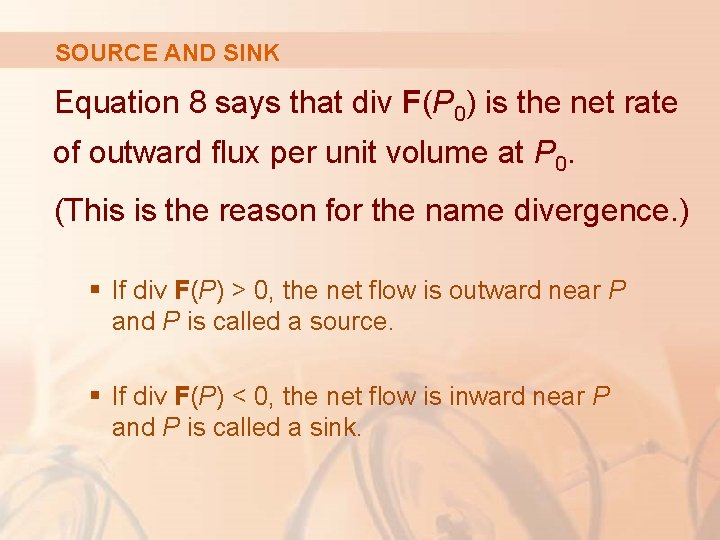SOURCE AND SINK Equation 8 says that div F(P 0) is the net rate of outward flux per unit volume at P 0. (This is the reason for the name divergence. ) § If div F(P) > 0, the net flow is outward near P and P is called a source. § If div F(P) < 0, the net flow is inward near P and P is called a sink.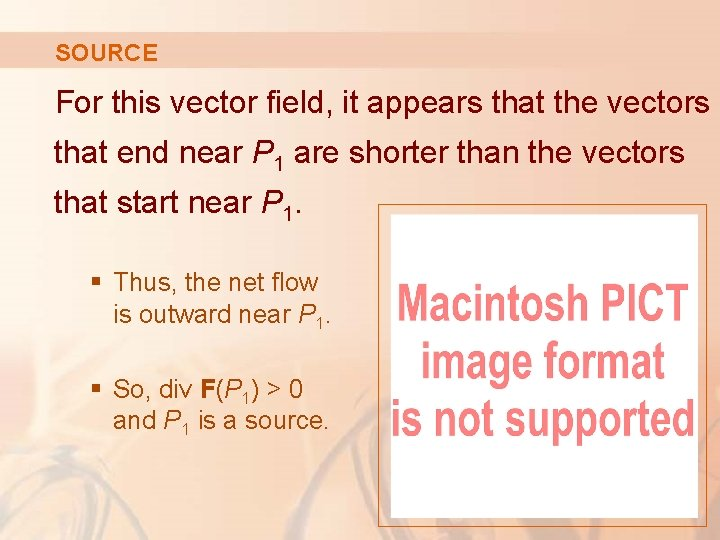SOURCE For this vector field, it appears that the vectors that end near P 1 are shorter than the vectors that start near P 1. § Thus, the net flow is outward near P 1. § So, div F(P 1) > 0 and P 1 is a source.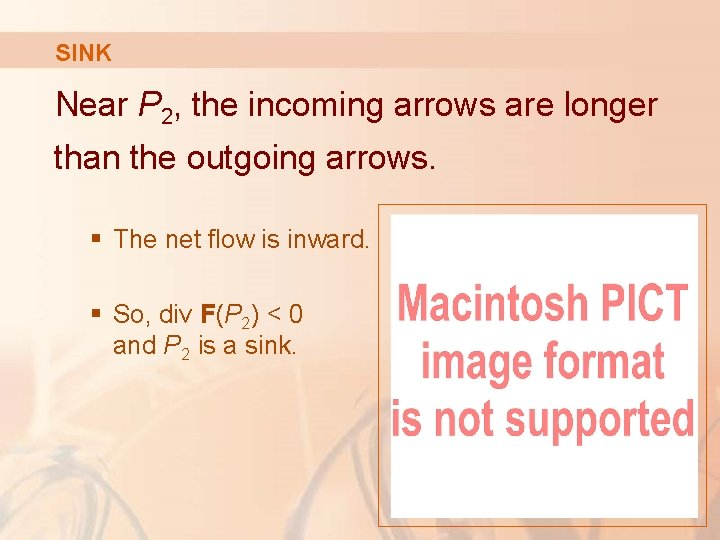SINK Near P 2, the incoming arrows are longer than the outgoing arrows. § The net flow is inward. § So, div F(P 2) < 0 and P 2 is a sink.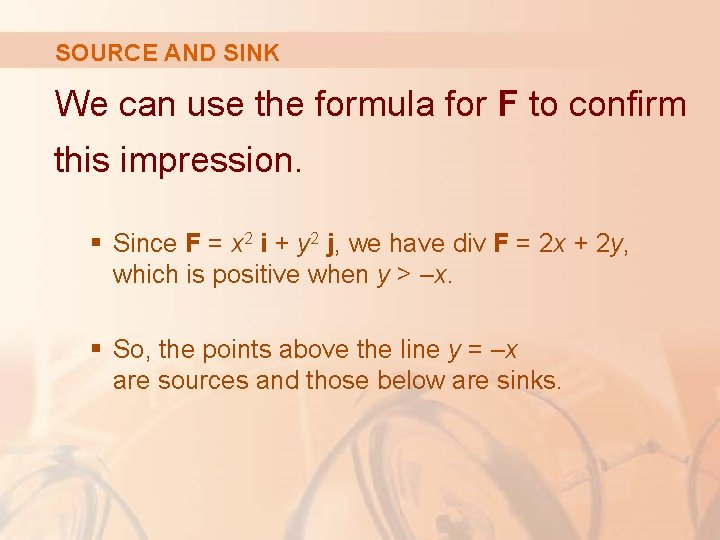SOURCE AND SINK We can use the formula for F to confirm this impression. § Since F = x 2 i + y 2 j, we have div F = 2 x + 2 y, which is positive when y > –x. § So, the points above the line y = –x are sources and those below are sinks.